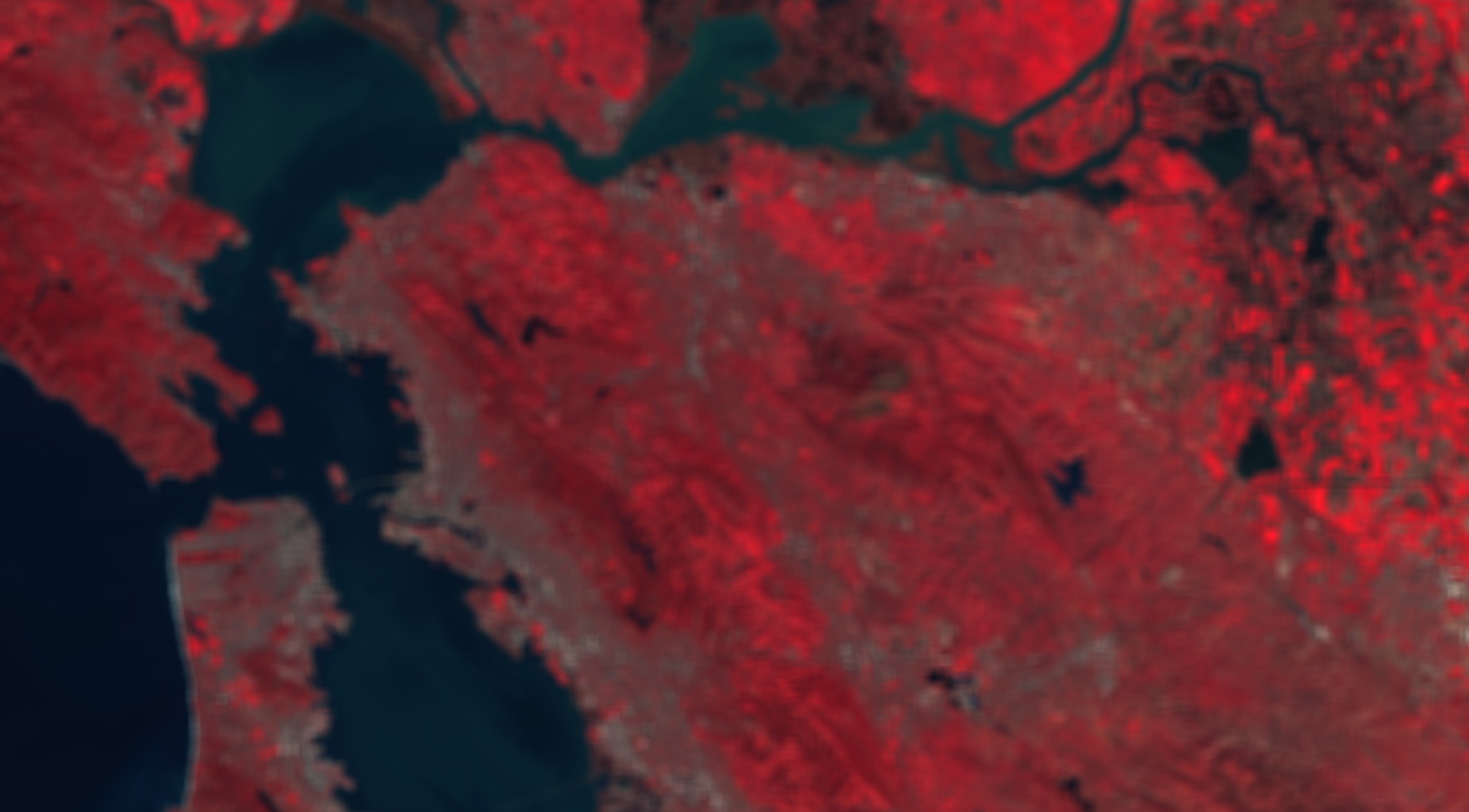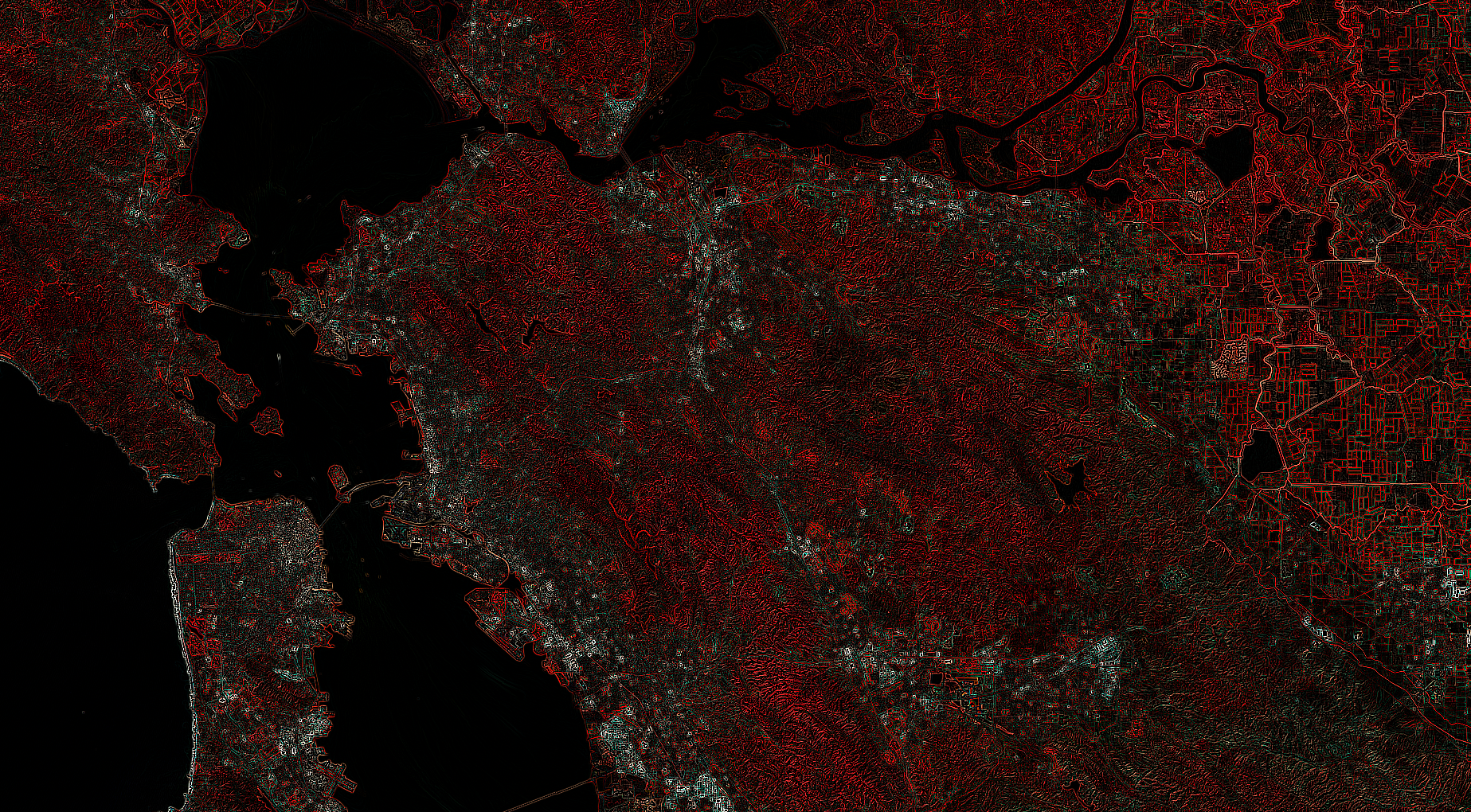# Convolutions

To perform linear convolutions on images, use `image.convolve()`. The only argument to convolve is an `ee.Kernel` which is specified by a shape and the weights in the kernel. Each pixel of the image output by `convolve()` is the linear combination of the kernel values and the input image pixels covered by the kernel. The kernels are applied to each band individually. For example, you might want to use a low-pass (smoothing) kernel to remove high-frequency information. The following illustrates a 15x15 low-pass kernel applied to a Landsat 8 image:

### Code Editor (JavaScript)

```// Load and display an image.
var image = ee.Image('LANDSAT/LC08/C02/T1_TOA/LC08_044034_20140318');
Map.setCenter(-121.9785, 37.8694, 11);
Map.addLayer(image, {bands: ['B5', 'B4', 'B3'], max: 0.5}, 'input image');

// Define a boxcar or low-pass kernel.
var boxcar = ee.Kernel.square({
radius: 7, units: 'pixels', normalize: true
});

// Smooth the image by convolving with the boxcar kernel.
var smooth = image.convolve(boxcar);
Map.addLayer(smooth, {bands: ['B5', 'B4', 'B3'], max: 0.5}, 'smoothed');```

The output of convolution with the low-pass filter should look something like Figure 1. Observe that the arguments to the kernel determine its size and coefficients. Specifically, with the `units` parameter set to pixels, the `radius` parameter specifies the number of pixels from the center that the kernel will cover. If `normalize` is set to true, the kernel coefficients will sum to one. If the `magnitude` parameter is set, the kernel coefficients will be multiplied by the magnitude (if `normalize` is also true, the coefficients will sum to `magnitude`). If there is a negative value in any of the kernel coefficients, setting `normalize` to true will make the coefficients sum to zero.Figure 1. Landsat 8 image convolved with a smoothing kernel. San Francisco Bay area, California, USA.

Use other kernels to achieve the desired image processing effect. This example uses a Laplacian kernel for isotropic edge detection:

### Code Editor (JavaScript)

```// Define a Laplacian, or edge-detection kernel.
var laplacian = ee.Kernel.laplacian8({ normalize: false });

// Apply the edge-detection kernel.
var edgy = image.convolve(laplacian);
{bands: ['B5', 'B4', 'B3'], max: 0.5, format: 'png'},
'edges');```

Note the format specifier in the visualization parameters. Earth Engine sends display tiles to the Code Editor in JPEG format for efficiency, however edge tiles are sent in PNG format to handle transparency of pixels outside the image boundary. When a visual discontinuity results, setting the format to PNG results in a consistent display. The result of convolving with the Laplacian edge detection kernel should look something like Figure 2.Figure 2. Landsat 8 image convolved with a Laplacian edge detection kernel. San Francisco Bay area, California, USA.

There are also anisotropic edge detection kernels (e.g. Sobel, Prewitt, Roberts), the direction of which can be changed with `kernel.rotate()`. Other low pass kernels include a Gaussian kernel and kernels of various shape with uniform weights. To create kernels with arbitrarily defined weights and shape, use `ee.Kernel.fixed()`. For example, this code creates a 9x9 kernel of 1’s with a zero in the middle:

### Code Editor (JavaScript)

```// Create a list of weights for a 9x9 kernel.
var row = [1, 1, 1, 1, 1, 1, 1, 1, 1];
// The center of the kernel is zero.
var centerRow = [1, 1, 1, 1, 0, 1, 1, 1, 1];
// Assemble a list of lists: the 9x9 kernel weights as a 2-D matrix.
var rows = [row, row, row, row, centerRow, row, row, row, row];
// Create the kernel from the weights.
var kernel = ee.Kernel.fixed(9, 9, rows, -4, -4, false);
print(kernel);```
[{ "type": "thumb-down", "id": "missingTheInformationINeed", "label":"Missing the information I need" },{ "type": "thumb-down", "id": "tooComplicatedTooManySteps", "label":"Too complicated / too many steps" },{ "type": "thumb-down", "id": "outOfDate", "label":"Out of date" },{ "type": "thumb-down", "id": "samplesCodeIssue", "label":"Samples / code issue" },{ "type": "thumb-down", "id": "otherDown", "label":"Other" }]
[{ "type": "thumb-up", "id": "easyToUnderstand", "label":"Easy to understand" },{ "type": "thumb-up", "id": "solvedMyProblem", "label":"Solved my problem" },{ "type": "thumb-up", "id": "otherUp", "label":"Other" }]